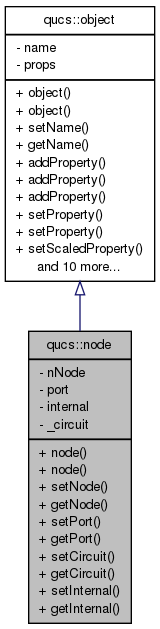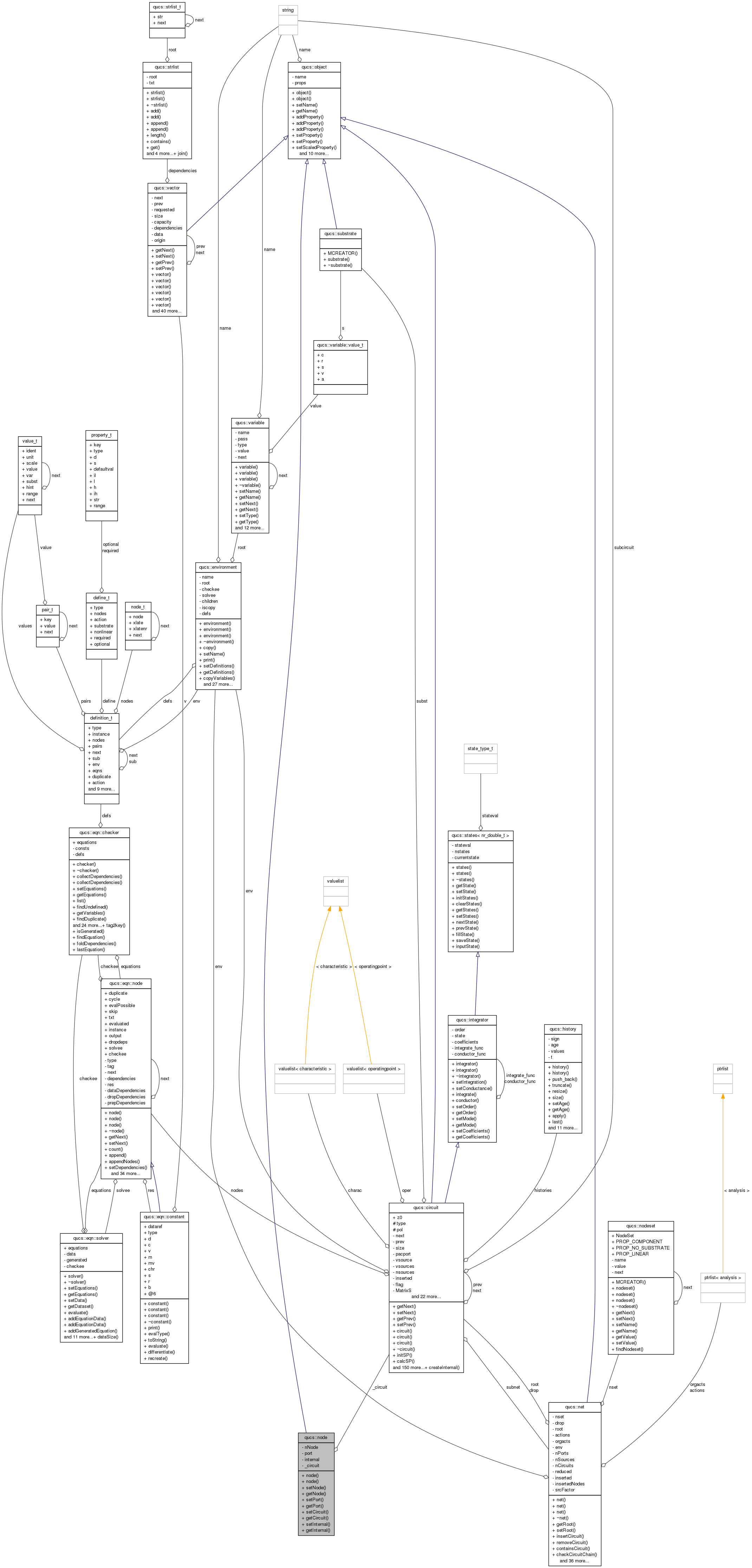Qucs-core  0.0.19
qucs::node Class Reference

`#include <node.h>`

Inheritance diagram for qucs::node:[legend]
Collaboration diagram for qucs::node:[legend]

Public Member Functions

node ()
Constructor creates an unnamed instance of the node class.
node (char *const n)
Constructor creates a named instance of the node class.
void setNode (const int n)
Sets the unique number of this node.
int getNode (void) const
Returns the unique number of this node.
void setPort (const int p)
Sets the port number of this node.
int getPort (void) const
Returns the port number of this node.
void setCircuit (circuit *const c)
Sets this node's circuit.
circuitgetCircuit (void) const
void setInternal (int i)
int getInternal (void)

int nNode
int port
int internal
circuit_circuit

Detailed Description

Definition at line 32 of file node.h.

Constructor & Destructor Documentation

 qucs::node::node ( ) ` [inline]`

Constructor creates an unnamed instance of the node class.

Definition at line 36 of file node.h.

 qucs::node::node ( char *const n ) ` [inline]`

Constructor creates a named instance of the node class.

Definition at line 38 of file node.h.

Member Function Documentation

 circuit* qucs::node::getCircuit ( void ) const` [inline]`

Definition at line 49 of file node.h.

 int qucs::node::getInternal ( void ) ` [inline]`

Definition at line 51 of file node.h.

 int qucs::node::getNode ( void ) const` [inline]`

Returns the unique number of this node.

Definition at line 42 of file node.h.

 int qucs::node::getPort ( void ) const` [inline]`

Returns the port number of this node.

Definition at line 46 of file node.h.

 void qucs::node::setCircuit ( circuit *const c ) ` [inline]`

Sets this node's circuit.

Definition at line 48 of file node.h.

 void qucs::node::setInternal ( int i ) ` [inline]`

Definition at line 50 of file node.h.

 void qucs::node::setNode ( const int n ) ` [inline]`

Sets the unique number of this node.

Definition at line 40 of file node.h.

 void qucs::node::setPort ( const int p ) ` [inline]`

Sets the port number of this node.

Definition at line 44 of file node.h.

Field Documentation

 circuit* qucs::node::_circuit` [private]`

Definition at line 57 of file node.h.

 int qucs::node::internal` [private]`

Definition at line 56 of file node.h.

 int qucs::node::nNode` [private]`

Definition at line 54 of file node.h.

 int qucs::node::port` [private]`

Definition at line 55 of file node.h.

The documentation for this class was generated from the following file: